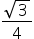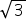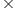Maths-
General
Easy

Question

# A star is made up of 4 equilateral triangles and a square. If the sides of the triangles are 8 units, what is the surface area of the star ?Hint:

## The correct answer is: 174.85 sq. units

### It is given that side of equilateral triangle = 8 unitsArea of equilateral triangle =a2=82  = 16square unitsSince there are 4 triangles, so area of four equilateral triangles= 416= 64sq. units= 110.85 sq. unitsIn the figure, length of the side of square = 8 unitsNow, Area of square = (side)2 = 82 = 64 sq. unitsSurface Area of the star= Area of 4 equilateral triangles + Area of the square=  110.85 + 64 = 174.85 sq. units#### With Turito Foundation.#### Get an Expert Advice From Turito.